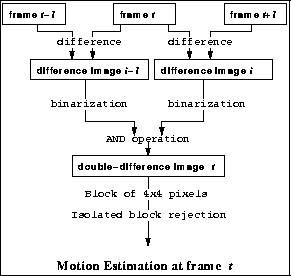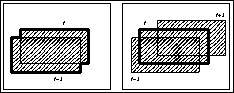Next: Human Model Up: No Title Previous: Introduction

# Double-difference image

We propose to use a double-difference image to get motion regions from video frames and estimate a pose partially on the region where the motion regions is detected. The motion region is a region where a pixel value changes. A double-difference image is obtained by AND operation between successive two difference images.

It is assumed that the video frames do not include any moving object except for a human body.

We make a double-difference image from three successive frames in an video stream(Figure 1). First, we generate two difference images from corresponding two successive images ('t-1' and 't', 't' and 't+1'). Then we binarize the difference images and execute AND operation on these two images. We call a resultant binary image a double-difference image.

As a double-difference image is a product of two difference images, it tends to include isolated noise pixels. These pixels disturbs motion estimation described later in Section 4. Therefore, each 4 by 4 pixels in the double-difference image is grouped into one square block. A block is marked true if more than half of the pixels in the block is true. This process not only prevents noise but also reduces the computation cost in the image processing. Then the system removes isolated blocks to get rid of slight changes in the video frames. The motion regions consists of the pixels whose value is true in the remained blocks.Figure 1: Double-difference Image Generation

A double-difference image has two good features. One is that motion regions on the double-difference image keeps the shape of the human body at time 't'. Regions on a normal difference image do not express the shape of the object because it is a mixture of the object shape on the image plane at time 't-1' and that at time 't'. For example, consider a rectangle object transition in Figure 2. In the left, extracted shape is a combined contour (thick line) of that of time t-1 and time t. The right figure shows a double-difference image and the AND operation keeps the original shape at time t.

The other feature is that it is easy to detect whether the current frame contains motion information or not. If motion regions on a double-difference image are small or do not exist, it indicates that the human body stands still and it is no need to estimate the pose in that frame(Figure 3).Figure 2: Extracted Shape on Difference ImagesNext: Human Model Up: No Title Previous: Introduction

Yoshinari Kameda
Mon Apr 7 22:34:43 JST 1997